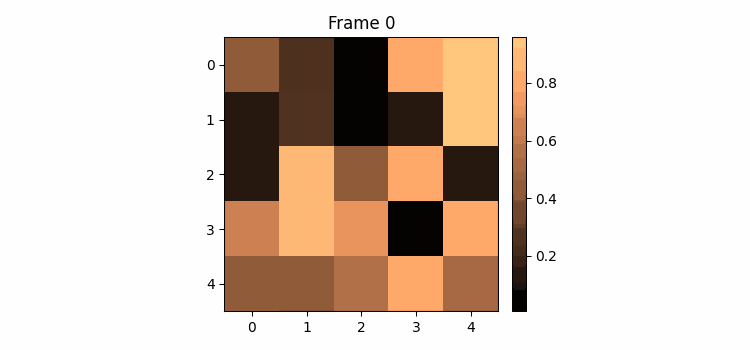# How to animate the colorbar in Matplotlib?

To animate the colorbar in matplotlib, we can take the following steps −

• Set the figure size and adjust the padding between and around the subplots.

• Create a new figure or activate an existing figure.

• Add an '~.axes.Axes' to the figure as part of a subplot arrangement.

• Instantiate Divider based on the pre-existing axes, i.e., ax object and return a new axis locator for the specified cell.

• Create an axes at the given *position* with the same height (or width) of the main axes.

• Create random data using numpy.

• Use imshow() method to plot random data.

• Set the title of the plot.

• Instantiate the list of colormaps.

• To animate the colorbar, use animate() method.

• To display the figure, use show() method.

## Example

import numpy as np
import matplotlib.pyplot as plt
import matplotlib.animation as animation
from mpl_toolkits.axes_grid1 import make_axes_locatable

plt.rcParams["figure.figsize"] = [7.50, 3.50]
plt.rcParams["figure.autolayout"] = True

fig = plt.figure()

div = make_axes_locatable(ax)
cax = div.append_axes('right', '5%', '5%')
data = np.random.rand(5, 5)
im = ax.imshow(data)
cb = fig.colorbar(im, cax=cax)
tx = ax.set_title('Frame 0')
cmap = ["copper", 'RdBu_r', 'Oranges', 'cividis', 'hot', 'plasma']

def animate(i):
cax.cla()
data = np.random.rand(5, 5)
im = ax.imshow(data, cmap=cmap[i%len(cmap)])
fig.colorbar(im, cax=cax)
tx.set_text('Frame {0}'.format(i))
ani = animation.FuncAnimation(fig, animate, frames=10)

plt.show()

## Output